# [paper notes] lsnet: extreme light weight Siamese network for change detection in remote sensing image

2022-05-15 07:20:49

# The paperThesis title ：LSNET: EXTREMELY LIGHT-WEIGHT SIAMESE NETWORK FOR CHANGE DETECTIONOF REMOTE SENSING IMAGE

The delivery ：CVPR 2022

The idea of this paper is relatively simple , Mainly made two modifications , The first is the lightweight of the backbone network , use CGB Modules build twin lightweight backbone networks ; On the other hand, it is the improvement of pyramid feature fusion , stay denseFPN Improve on the basis of , Remove redundant connections , Increase the bottom-up fusion path . The reason why the amount of parameters and calculation of the model are greatly reduced is the lightweight of the backbone network , The depth separable convolution is used to replace the ordinary convolution operation .

# experimental resultOfficial training parameters ：

``````{
"patch_size": 256,
"augmentation": true,
"num_gpus": 1,
"num_workers": 8,
"num_channel": 3,
"EF": false,
"epochs": 101,
"batch_size": 12,
"learning_rate": 1e-3,
"model_name": "denseFPN",
"loss_function": "contra_hybrid",
"dataset_dir": "data/Real/subset/",
"weight_dir": "./outputs/",
"log_dir": "./log/"
}``````

# Abstract

Twin networks have gradually become remote sensing images （remote sensing image,RSI） The mainstream of change detection . But with the structure 、 Complexity of modules and training process , The model is becoming more and more complex , Difficult to apply in practice .

this paper , A method for RSI Ultra lightweight twin network for change detection （Light-Weight Siamese Network,LSNet）, The standard convolution is replaced by deep separable convolution and void convolution , And remove redundant dense connections , Only the effective feature flow is retained in the twin feature fusion , It greatly reduces the parameters and calculation . stay CDD On dataset , Compared with the first model ,LSNet The parameters and calculation are reduced respectively 90.35% and 91.34%, The accuracy is only reduced 1.5%.

# Introduction

Conventional RSI The change detection method depends on artificial features and time-consuming pre and post-processing , It is difficult to distinguish between semantic change and background noise .

The image pair can be directly input into the twin convolution network , No preprocessing is required , Depending on end-to-end supervised learning, we can separate semantic change regions and invariant regions .• This paper presents a lightweight twin network LSNet, It's very efficient , Pictured 1. The network backbone adopts the context guidance module （Context Guide Block,CGB） structure , The core components of the module are deep separable hole convolution and global feature aggregation . Compared to using ResNet-50 As the backbone ,LSNet The parameter quantity and calculation quantity of the trunk are only the original 3.97% and 32.56%.
• A characteristic pyramid of difference network is proposed （diffFPN） To carry out progressive feature difference extraction and resolution restoration （ Eliminate redundant connections while maintaining feature flow ）, Finally, the changing image region is separated from the constant image region .

# Method

LSNet： Including a twin backbone （LightSiamese Backbone） And a differential characteristic pyramid network （diffFPN）. The backbone uses the context guidance module （CGB） structure ,diffFPN For effective twin feature pair fusion .## Light-Siamese backbone

Images T1 and T2 Through the twin network backbone with shared weight , The backbone network consists of 4 Composed of two composite layers （ From top to bottom , The composite layer consists of 3/3/8/12 individual CGB Module composition ）, Every CGB Equivalent to two levels , So there is 4 Group feature output , in total 52 layer .

Basic components （Context Guide Block）CGB Pictured 2 On the right . Input X After parallel expansion （ inflation ） Convolution , To get different ranges （ Feel the field ） Local context information . The expanded convolution is calculated in a deeply separable manner , That is, all channels are grouped , Convolution operates only in a separate group .（ Depth separates the convolution , It can greatly reduce the amount of calculation , But there is an upper limit to speed , The bottleneck of computing power lies in the access bandwidth ）Channel interaction and global information extraction .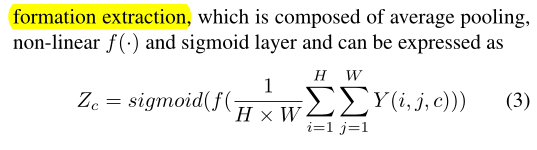## Differential feature pyramid network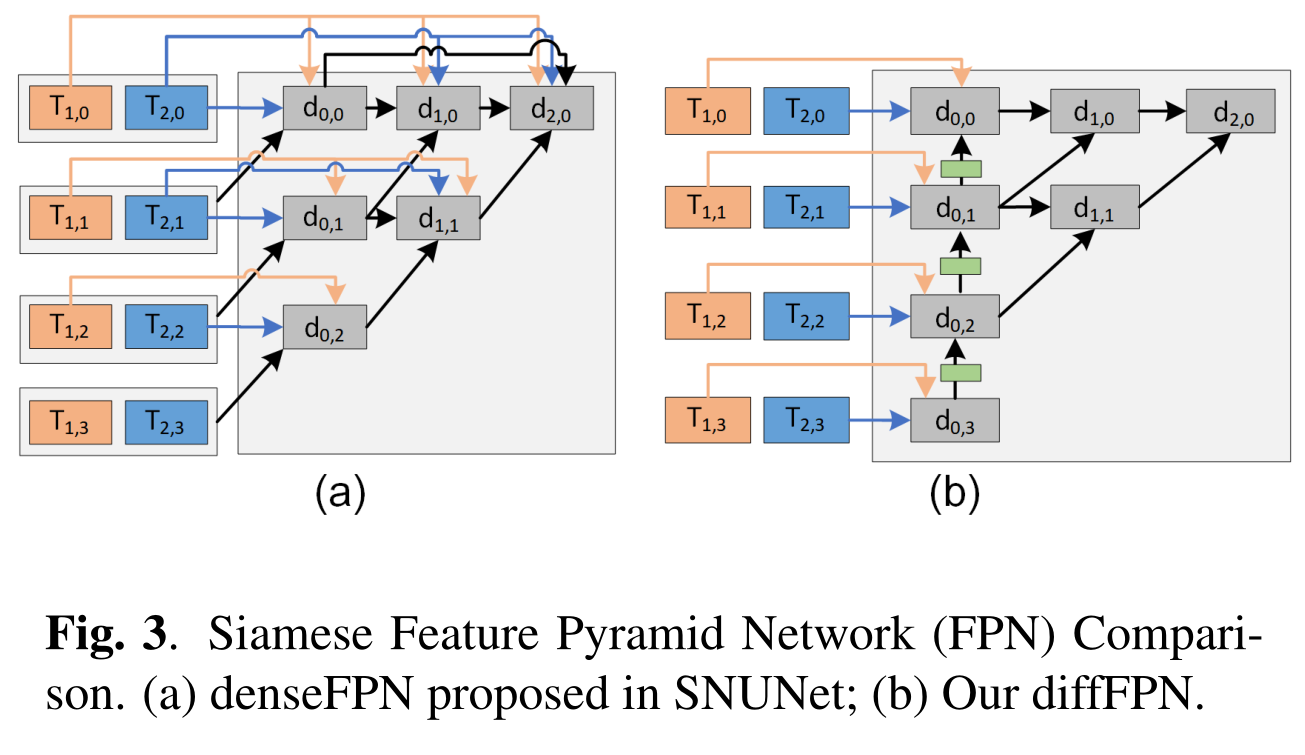SUNNet A pyramid feature fusion method with dense connection is proposed , chart 3（a） Shown ,such denseFPN Structure exists 2 A question ：

• Redundant connections .（T_1,0、T_2,0 Such shallow features are repeatedly input into d_1,0、d_2,0、d_3,0 in , inefficiency ）
• Unreasonable characteristic flow .（denseFPN in , Output layer d_0,0 and d_1,0 Contains incomplete features from the backbone ）

therefore , The paper proposes diffFPPN structure ,  Remove redundant connections , Added a bottom-up fusion path , Make the three output layers include the complete backbone network features .# Experiment and Results

## Dataset and evaluation metrics

Data sets ：CDD

Common indicators ： precision、recall、F1-score、overall accuracy

Quantitative index ：F1-G、F1-F （ The quantization unit parameters and the amount of calculation are right F1 The impact of scores ）,F1-Eff（ Evaluate the overall efficiency of the model ）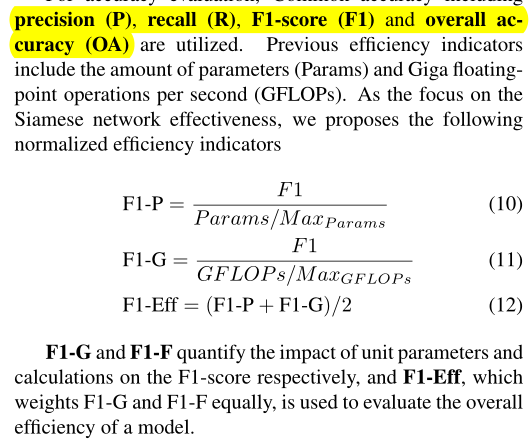## Accuracy and efficiency comparison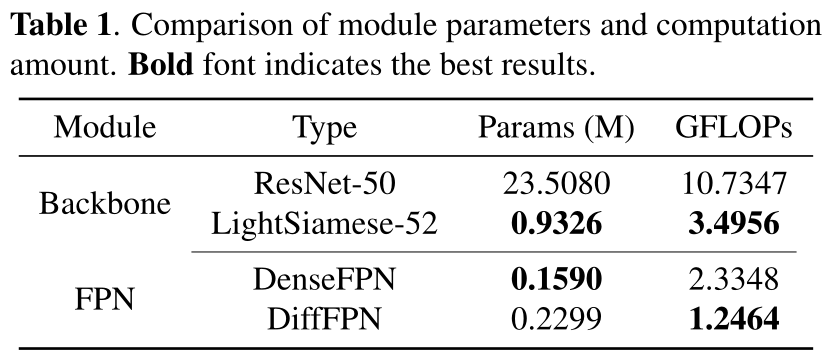Comparison of parameters and calculation between the two modules （CDD Data sets ）, As can be seen from the table ,

• comparison ResNet-50,LightSiamese-52 The parameter quantity and calculation quantity of the trunk are only the original 1/25 and 1/3.
• denseFPN There is irrationality of characteristic flow in the structure of ,diffFPN Then only lift 0.0709M When the parameter quantity of , The amount of calculation is reduced 1.0884GFLOPs, Cut by more than half .There are many ways to CDD Performance comparison in data set ,LSNet The performance indexes of the method are still ok, Top three .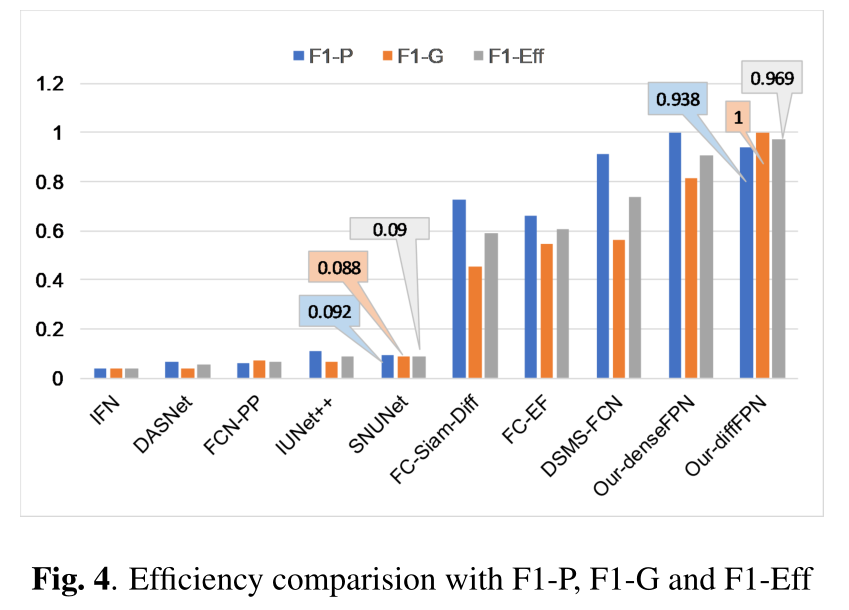Efficiency comparison of various methods , Visible use diffFPN The method has the highest F1-P and F1-G.

Combine tables 2 Sum graph 3, And SNUNet comparison ,LSNet The parameters and calculation are reduced respectively 90.35% and 91.34%, Accuracy only decreases 1.5%.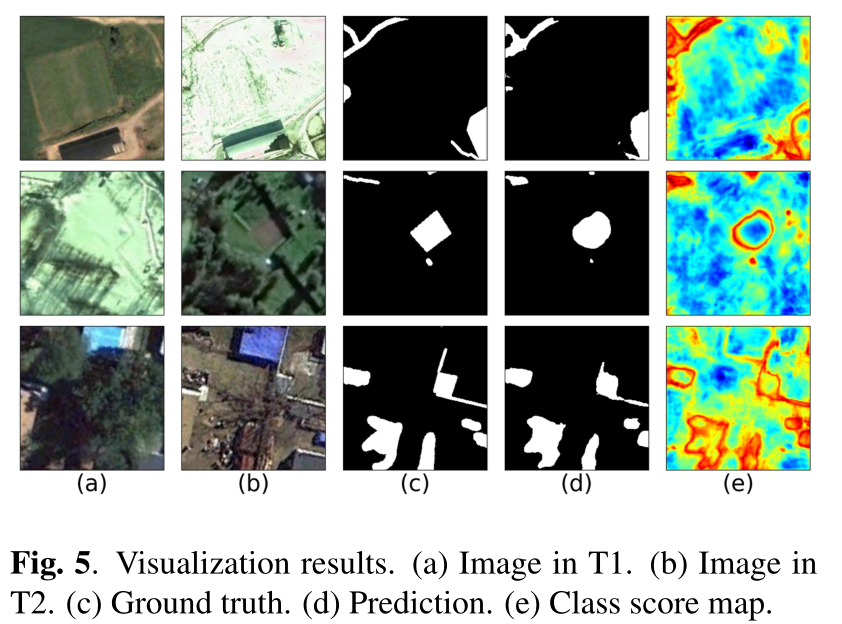LSNet Visual results of . The results are relatively accurate , But the edge details need to be further refined .（e） It can be seen that , The edge of the change area has a higher probability than the interior , It shows that the network uses the structure of the region as the identification feature , It improves its robustness to color and texture changes .

# Conclusion

In order to effectively detect RSI change , A lightweight twin network is proposed , The network has a context guided module （CGB） Build a lightweight twin trunk （LightSiamese Backbone） And feature pair fusion module （diffFPN）. In challenging CCD The results on the data set show that , Compared with other mainstream methods , This method obtains competitive results with limited parameters and calculation , Proved its validity .

# Core code

## Context Guide Block

``````class ContextGuidedBlock(nn.Module):
"""Context Guided Block for CGNet.

This class consists of four components: local feature extractor,
surrounding feature extractor, joint feature extractor and global
context extractor.

Args:
in_channels (int): Number of input feature channels.
out_channels (int): Number of output feature channels.
dilation (int): Dilation rate for surrounding context extractor.
Default: 2.
reduction (int): Reduction for global context extractor. Default: 16.
skip_connect (bool): Add input to output or not. Default: True.
downsample (bool): Downsample the input to 1/2 or not. Default: False.
conv_cfg (dict): Config dict for convolution layer.
Default: None, which means using conv2d.
norm_cfg (dict): Config dict for normalization layer.
act_cfg (dict): Config dict for activation layer.
Default: dict(type='PReLU').
with_cp (bool): Use checkpoint or not. Using checkpoint will save some
memory while slowing down the training speed. Default: False.
"""

def __init__(self,
in_channels,
out_channels,
dilation=2,
reduction=16,
skip_connect=True,
downsample=False,
conv_cfg=None,
act_cfg=dict(type='PReLU'),
with_cp=False):
super(ContextGuidedBlock, self).__init__()
self.with_cp = with_cp
self.downsample = downsample

# channels = out_channels if downsample else out_channels // 2
channels = out_channels // 2
if 'type' in act_cfg and act_cfg['type'] == 'PReLU':
act_cfg['num_parameters'] = channels
kernel_size = 3 if downsample else 1
stride = 2 if downsample else 1
padding = (kernel_size - 1) // 2
# self.channel_shuffle = ChannelShuffle(2 if in_channels==in_channels//2*2 else in_channels)
self.conv1x1 = nn.Sequential(
build_norm_layer(channels),
nn.PReLU(num_parameters=channels)
)

self.f_loc = nn.Conv2d(channels, channels, kernel_size=3,

self.f_sur = nn.Conv2d(channels, channels, kernel_size=3, padding=dilation,
dilation=dilation, groups=channels, bias=False)

self.bn = build_norm_layer(2 * channels)
self.activate = nn.PReLU(2 * channels)

# original bottleneck in CGNet: A light weight context guided network for segmantic segmentation
# is removed for saving computation amount
# if downsample:
#     self.bottleneck = build_conv_layer(
#         conv_cfg,
#         2 * channels,
#         out_channels,
#         kernel_size=1,
#         bias=False)

self.skip_connect = skip_connect and not downsample
self.f_glo = GlobalContextExtractor(out_channels, reduction, with_cp)
# self.f_glo = CoordAtt(out_channels,out_channels,groups=reduction)

def forward(self, x):

def _inner_forward(x):
# x = self.channel_shuffle(x)
out = self.conv1x1(x)
loc = self.f_loc(out)
sur = self.f_sur(out)

joi_feat = torch.cat([loc, sur], 1)  # the joint feature
joi_feat = self.bn(joi_feat)
joi_feat = self.activate(joi_feat)
if self.downsample:
pass
# joi_feat = self.bottleneck(joi_feat)  # channel = out_channels
# f_glo is employed to refine the joint feature
out = self.f_glo(joi_feat)

if self.skip_connect:
return x + out
else:
return out

return _inner_forward(x)

def cgblock(in_ch, out_ch, dilation=2, reduction=8, skip_connect=False):
return nn.Sequential(
ContextGuidedBlock(in_ch, out_ch,
dilation=dilation,
reduction=reduction,
downsample=False,
skip_connect=skip_connect))
``````

## light_siamese_backbone

``````class light_siamese_backbone(nn.Module):
def __init__(self, in_ch=None, num_blocks=None, cur_channels=None,
filters=None, dilations=None, reductions=None):
super(light_siamese_backbone, self).__init__()
norm_cfg = {'type': 'BN', 'eps': 0.001, 'requires_grad': True}
act_cfg = {'type': 'PReLU', 'num_parameters': 32}
self.inject_2x = InputInjection(1)  # down-sample for Input, factor=2
self.inject_4x = InputInjection(2)  # down-sample for Input, factor=4
# stage 0
self.stem = nn.ModuleList()
for i in range(num_blocks):
self.stem.append(
ContextGuidedBlock(
cur_channels, filters,
dilations, reductions,
skip_connect=(i != 0),
downsample=False,
norm_cfg=norm_cfg,
act_cfg=act_cfg)  # CG block
)
cur_channels = filters

cur_channels += in_ch
self.norm_prelu_0 = nn.Sequential(
build_norm_layer(cur_channels),
nn.PReLU(cur_channels))

# stage 1
self.level1 = nn.ModuleList()
for i in range(num_blocks):
self.level1.append(
ContextGuidedBlock(
cur_channels if i == 0 else filters,
filters, dilations, reductions,
downsample=(i == 0),
norm_cfg=norm_cfg,
act_cfg=act_cfg))  # CG block

cur_channels = 2 * filters + in_ch
self.norm_prelu_1 = nn.Sequential(
build_norm_layer(cur_channels),
nn.PReLU(cur_channels))

# stage 2
self.level2 = nn.ModuleList()
for i in range(num_blocks):
self.level2.append(
ContextGuidedBlock(
cur_channels if i == 0 else filters,
filters, dilations, reductions,
downsample=(i == 0),
norm_cfg=norm_cfg,
act_cfg=act_cfg))  # CG block

cur_channels = 2 * filters
self.norm_prelu_2 = nn.Sequential(
build_norm_layer(cur_channels),
nn.PReLU(cur_channels))

# stage 3
self.level3 = nn.ModuleList()
for i in range(num_blocks):
self.level3.append(
ContextGuidedBlock(
cur_channels if i == 0 else filters,
filters, dilations, reductions,
downsample=(i == 0),
norm_cfg=norm_cfg,
act_cfg=act_cfg))  # CG block

cur_channels = 2 * filters
self.norm_prelu_3 = nn.Sequential(
build_norm_layer(cur_channels),
nn.PReLU(cur_channels))

def forward(self, x):
# x = torch.cat([xA, xB], dim=0)
# stage 0
inp_2x = x  # self.inject_2x(x)
inp_4x = self.inject_2x(x)
for layer in self.stem:
x = layer(x)
x = self.norm_prelu_0(torch.cat([x, inp_2x], 1))
x0_0A, x0_0B = x[:x.shape // 2, :, :, :], x[x.shape // 2:, :, :, :]

# stage 1
for i, layer in enumerate(self.level1):
x = layer(x)
if i == 0:
down1 = x
x = self.norm_prelu_1(torch.cat([x, down1, inp_4x], 1))
x1_0A, x1_0B = x[:x.shape // 2, :, :, :], x[x.shape // 2:, :, :, :]

# stage 2
for i, layer in enumerate(self.level2):
x = layer(x)
if i == 0:
down1 = x
x = self.norm_prelu_2(torch.cat([x, down1], 1))
x2_0A, x2_0B = x[:x.shape // 2, :, :, :], x[x.shape // 2:, :, :, :]

# stage 3
for i, layer in enumerate(self.level3):
x = layer(x)
if i == 0:
down1 = x
x = self.norm_prelu_3(torch.cat([x, down1], 1))
x3_0A, x3_0B = x[:x.shape // 2, :, :, :], x[x.shape // 2:, :, :, :]

return [x0_0A, x0_0B, x1_0A, x1_0B, x2_0A, x2_0B, x3_0A, x3_0B]

class InputInjection(nn.Module):
"""Downsampling module for CGNet."""

def __init__(self, num_downsampling):
super(InputInjection, self).__init__()
self.pool = nn.ModuleList()
for i in range(num_downsampling):

def forward(self, x):
for pool in self.pool:
x = pool(x)
return x

def build_norm_layer(ch):
layer = nn.BatchNorm2d(ch, eps=0.01)
for param in layer.parameters():
return layer
``````

## diffFPN

``````class diffFPN(nn.Module):
def __init__(self, cur_channels=None, mid_ch=None,
dilations=None, reductions=None,
bilinear=True):
super(diffFPN, self).__init__()
# lateral convs for unifing channels
self.lateral_convs = nn.ModuleList()
for i in range(4):
self.lateral_convs.append(
cgblock(cur_channels[i] * 2, mid_ch * 2 ** i, dilations[i], reductions[i])
)
# top_down_convs
self.top_down_convs = nn.ModuleList()
for i in range(3, 0, -1):
self.top_down_convs.append(
cgblock(mid_ch * 2 ** i, mid_ch * 2 ** (i - 1), dilation=dilations[i], reduction=reductions[i])
)

# diff convs
self.diff_convs = nn.ModuleList()
for i in range(3):
self.diff_convs.append(
cgblock(mid_ch * (3 * 2 ** i), mid_ch * 2 ** i, dilations[i], reductions[i])
)
for i in range(2):
self.diff_convs.append(
cgblock(mid_ch * (3 * 2 ** i), mid_ch * 2 ** i, dilations[i], reductions[i])
)
self.diff_convs.append(
cgblock(mid_ch * 3, mid_ch * 2,
dilation=dilations, reduction=reductions)
)
self.up2x = up(32, bilinear)

def forward(self, output):
tmp = [self.lateral_convs[i](torch.cat([output[i * 2], output[i * 2 + 1]], dim=1))
for i in range(4)]

# top_down_path
for i in range(3, 0, -1):
tmp[i - 1] += self.up2x(self.top_down_convs[3 - i](tmp[i]))

# x0_1
tmp = [self.diff_convs[i](torch.cat([tmp[i], self.up2x(tmp[i + 1])], dim=1)) for i in [0, 1, 2]]
x0_1 = tmp
# x0_2
tmp = [self.diff_convs[i](torch.cat([tmp[i - 3], self.up2x(tmp[i - 2])], dim=1)) for i in [3, 4]]
x0_2 = tmp
# x0_3
x0_3 = self.diff_convs(torch.cat([tmp, self.up2x(tmp)], dim=1))

return x0_1, x0_2, x0_3

``````

## LSNet_diffFPN

``````class LSNet_diffFPN(nn.Module):
# SNUNet-CD with ECAM
def __init__(self, in_ch=3, mid_ch=32, out_ch=2, bilinear=True):
super(LSNet_diffFPN, self).__init__()
torch.nn.Module.dump_patches = True

n1 = 32  # the initial number of channels of feature map
filters = (n1, n1 * 2, n1 * 4, n1 * 8, n1 * 16)
num_blocks = (3, 3, 8, 12)
dilations = (1, 2, 4, 8)
reductions = (4, 8, 16, 32)
cur_channels = [0, 0, 0, 0]
cur_channels = in_ch

self.backbone = light_siamese_backbone(in_ch=in_ch, num_blocks=num_blocks,
cur_channels=cur_channels,
filters=filters, dilations=dilations,
reductions=reductions)

self.FPN = diffFPN(cur_channels=cur_channels, mid_ch=mid_ch,
dilations=dilations, reductions=reductions, bilinear=bilinear)

for m in self.modules():
if isinstance(m, nn.Conv2d):
nn.init.kaiming_normal_(m.weight, mode='fan_out', nonlinearity='relu')
elif isinstance(m, (nn.BatchNorm2d, nn.GroupNorm)):
nn.init.constant_(m.weight, 1)
nn.init.constant_(m.bias, 0)

def forward(self, x, debug=False):

output = self.backbone(x)

x0_1, x0_2, x0_3 = self.FPN(output)